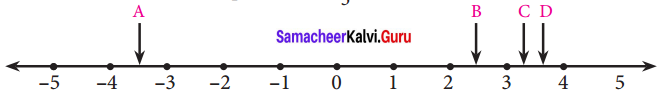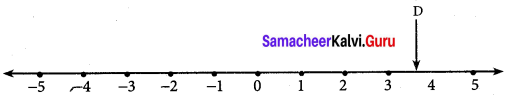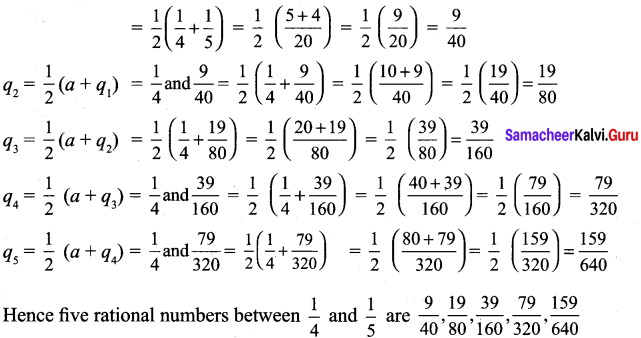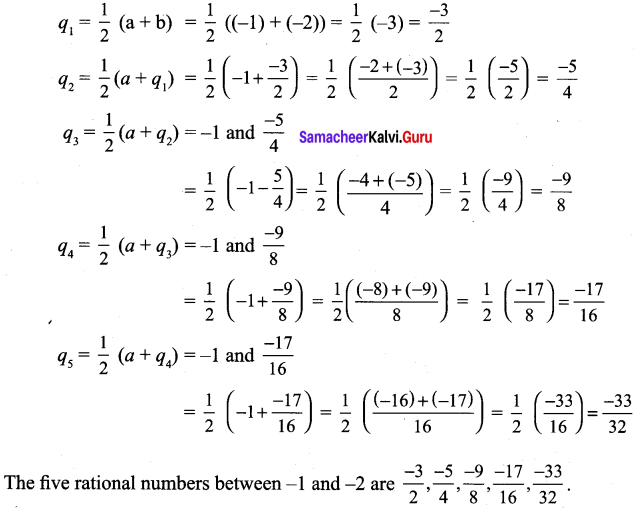## Tamilnadu Samacheer Kalvi 9th Maths Solutions Chapter 2 Real Numbers Ex 2.1

9th Maths Exercise 2.1 Samacheer Kalvi Question 1.
Which arrow best shows the position of $$\frac { 11 }{ 3 }$$ on the number line?Solution:
$$\frac { 11 }{ 3 }$$ = 3.666….. = 3.7 (nearly)
∴ D arrow best shows the position of $$\frac { 11 }{ 3 }$$ on the number line.9th Maths Real Numbers Exercise 2.1 Question 2.
Find any three rational numbers between $$\frac { -7 }{ 11 }$$ and $$\frac { 2 }{ 11 }$$.
Solution:
Three rational numbers between $$\frac { -7 }{ 11 }$$ and $$\frac { 2 }{ 11 }$$ are $$\frac { -6 }{ 11 }$$, $$\frac { -5 }{ 11 }$$, $$\frac { -4 }{ 11 }$$, ……, $$\frac { -1 }{ 11 }$$

9th Maths Exercise 2.1 Question 3.
Find any five rational numbers between
(i) $$\frac { 1 }{ 4 }$$ and $$\frac { 1 }{ 5 }$$
(ii) 0.1 and 0.11
(iii) -1 and -2
Solution:
(i) a = $$\frac { 1 }{ 4 }$$, b = $$\frac { 1 }{ 5 }$$
Let q1, q2, q3, q4 and q5 be five rational numbers, q1 = $$\frac { 1 }{ 2 }$$(a + b)(ii) The rational numbers between 0.1 and 0.11 are 0.101, 0.102, 0.103, …..0.109.
(iii) -1 and -2
Let q1, q2, q3, q4 and q5 be five rational numbers.